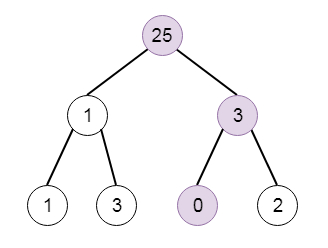# Smallest String Starting From Leaf in Python

Suppose we have the root of a binary tree, each node is containing a value from 0 to 25 , which are representing the letters 'a' to 'z': a value of 0 represents 'a', a value of 1 represents 'b', and so on. We have to search the lexicographically smallest string that starts at a leaf of this tree and finishes at the root. So if the tree is like −Then the output will be “adz” as the sequence is [0,3,25]

To solve this, we will follow these steps −

• Define a dfs traversal method as follows

• if node is not null, then

• insert node value as a character into A

• if node has no left and right child, then

• ans := minimum of ans and A elements as string in reversed form

• delete the last element from A

• return

• perform dfs(left of node, A)

• perform dfs(right of node, A)

• Delete last element from A

• return

• The actual method will be like −

• ans := “~”

• call dfs(root, an empty array as A)

• return ans

Let us see the following implementation to get better understanding −

## Example

Live Demo

class TreeNode:
def __init__(self, data, left = None, right = None):
self.data = data
self.left = left
self.right = right
def insert(temp,data):
que = []
que.append(temp)
while (len(que)):
temp = que
que.pop(0)
if (not temp.left):
if data is not None:
temp.left = TreeNode(data)
else:
temp.left = TreeNode(0)
break
else:
que.append(temp.left)
if (not temp.right):
if data is not None:
temp.right = TreeNode(data)
else:
temp.right = TreeNode(0)
break
else:
que.append(temp.right)
def make_tree(elements):
Tree = TreeNode(elements)
for element in elements[1:]:
insert(Tree, element)
return Tree
class Solution(object):
def smallestFromLeaf(self, root):
self.ans = "~"
self.dfs(root,[])
return self.ans
def dfs(self, node, A):
if node:
A.append(chr(node.data + ord('a')))
if not node.left and not node.right:
self.ans = min(self.ans, ''.join(reversed(A)))
A.pop()
return
self.dfs(node.left,A)
self.dfs(node.right,A)
A.pop()
return
root = make_tree([25,1,3,1,3,0,2])
ob = Solution()
print(ob.smallestFromLeaf(root))

## Input

[25,1,3,1,3,0,2]

## Output

adz